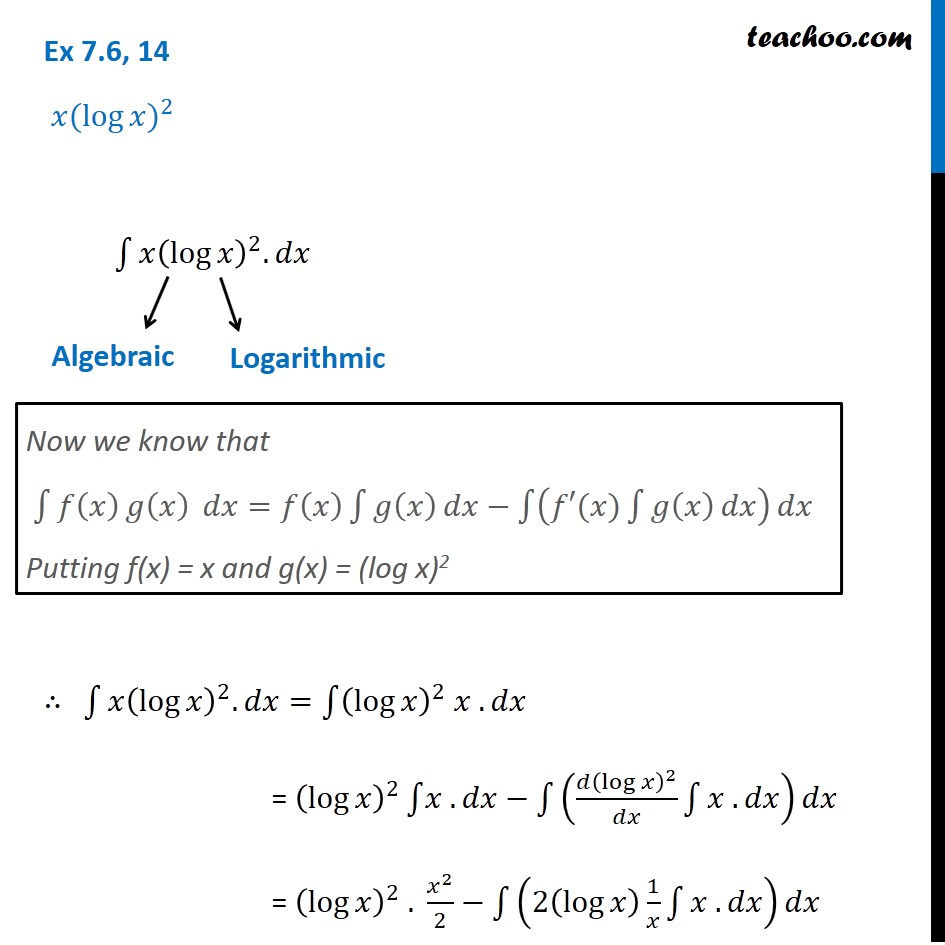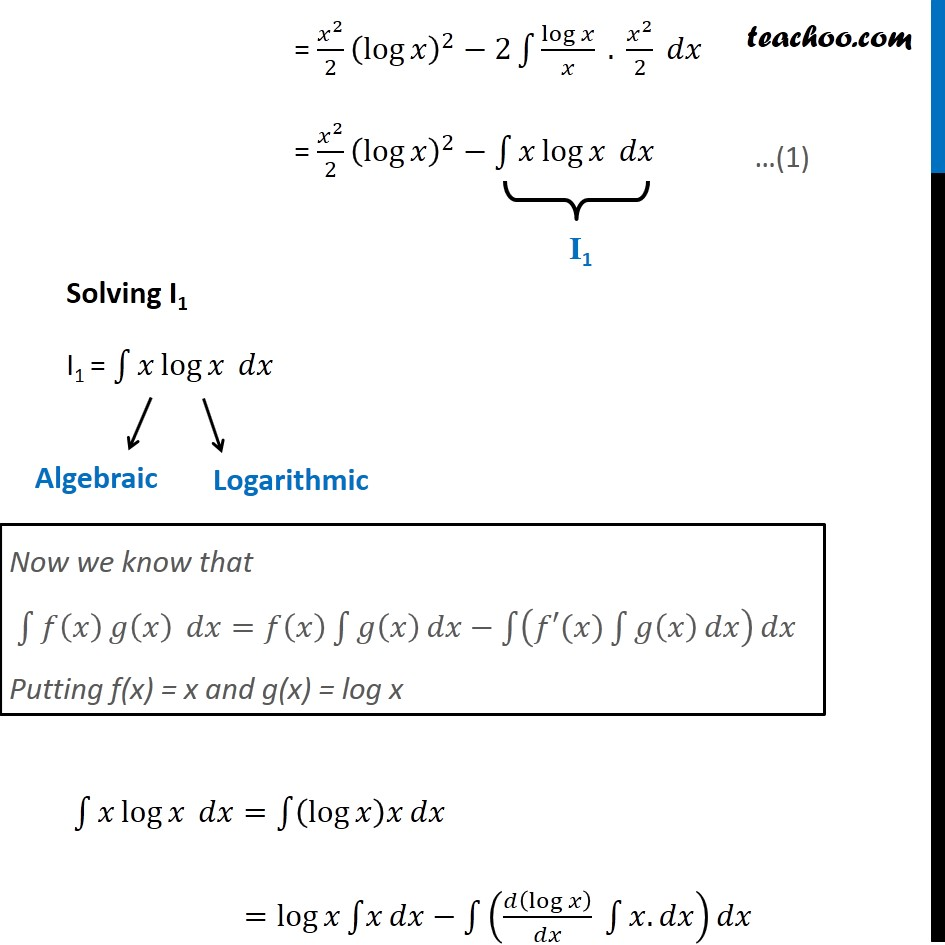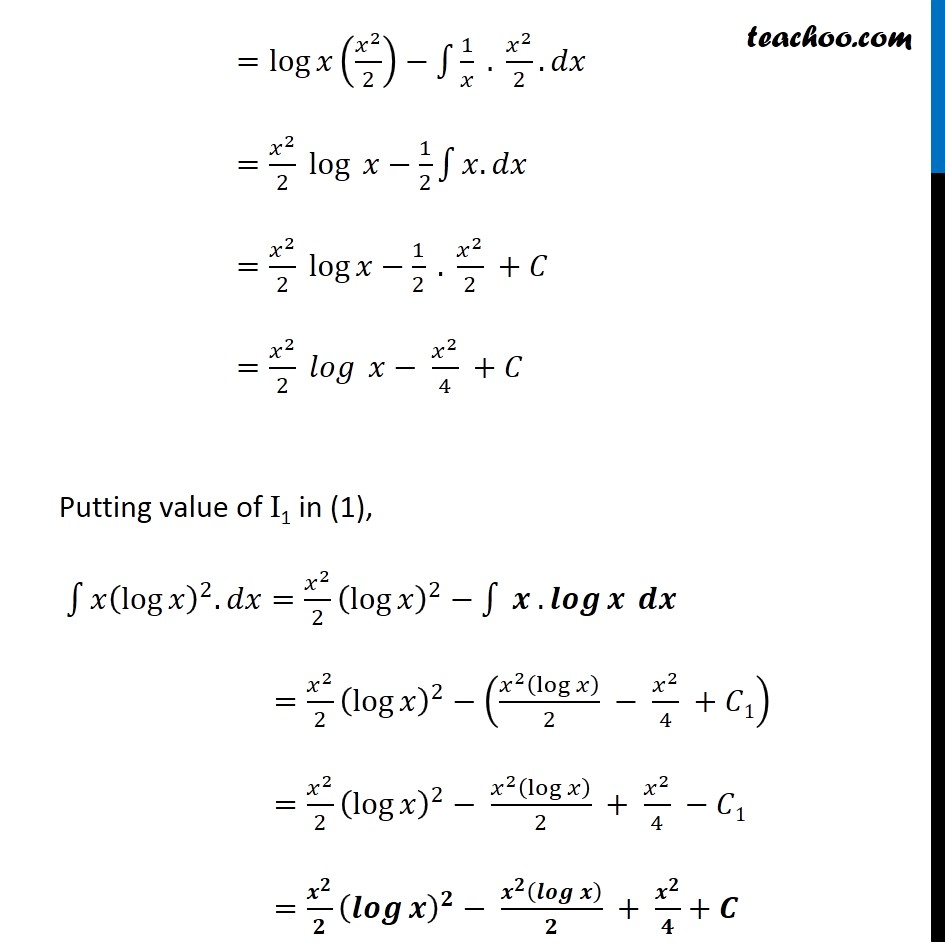Integration by parts

Chapter 7 Class 12 Integrals
Concept wiseLearn in your speed, with individual attention - Teachoo Maths 1-on-1 Class

### Transcript

Ex 7.6, 14 〖𝑥(log⁡𝑥)〗^2 ∫1▒〖𝑥(log⁡𝑥 )^2.𝑑𝑥 " " 〗 ∴ ∫1▒〖𝑥(log⁡𝑥 )^2.𝑑𝑥〗=∫1▒〖(log⁡𝑥 )^2 𝑥 .𝑑𝑥〗 = (log⁡𝑥 )^2 ∫1▒〖𝑥 .〗 𝑑𝑥−∫1▒((𝑑(log⁡𝑥 )^2)/𝑑𝑥 ∫1▒〖𝑥 .𝑑𝑥〗) 𝑑𝑥 = (log⁡𝑥 )^2 . 𝑥^2/2−∫1▒(2(log⁡𝑥 ) 1/𝑥 ∫1▒〖𝑥 .𝑑𝑥〗) 𝑑𝑥 Now we know that ∫1▒〖𝑓(𝑥) 𝑔⁡(𝑥) 〗 𝑑𝑥=𝑓(𝑥) ∫1▒𝑔(𝑥) 𝑑𝑥−∫1▒(𝑓′(𝑥)∫1▒𝑔(𝑥) 𝑑𝑥) 𝑑𝑥 Putting f(x) = x and g(x) = (log x)2 = 𝑥^2/2 (log⁡𝑥 )^2−2∫1▒〖log⁡𝑥/𝑥 . 𝑥^2/2〗 𝑑𝑥 = 𝑥^2/2 (log⁡𝑥 )^2−∫1▒〖𝑥 log⁡𝑥 〗 𝑑𝑥 Solving I1 I1 = ∫1▒〖𝑥 log⁡𝑥 〗 𝑑𝑥 ∫1▒〖𝑥 log⁡𝑥 〗 𝑑𝑥=∫1▒(log⁡𝑥 )𝑥 𝑑𝑥 =log⁡𝑥 ∫1▒𝑥 𝑑𝑥−∫1▒(𝑑(log⁡𝑥 )/𝑑𝑥 ∫1▒〖𝑥.𝑑𝑥〗)𝑑𝑥 Now we know that ∫1▒〖𝑓(𝑥) 𝑔⁡(𝑥) 〗 𝑑𝑥=𝑓(𝑥) ∫1▒𝑔(𝑥) 𝑑𝑥−∫1▒(𝑓′(𝑥)∫1▒𝑔(𝑥) 𝑑𝑥) 𝑑𝑥 Putting f(x) = x and g(x) = log x =log⁡𝑥 (𝑥^2/2)−∫1▒〖1/𝑥 . 𝑥^2/2. 𝑑𝑥〗 =〖𝑥^2/2 log〗⁡〖 𝑥〗−1/2 ∫1▒〖𝑥. 𝑑𝑥〗 =〖𝑥^2/2 log〗⁡𝑥−1/2 . 𝑥^2/2 +𝐶 =〖𝑥^2/2 𝑙𝑜𝑔〗⁡〖 𝑥〗− 𝑥^2/4 +𝐶 Putting value of I1 in (1), ∫1▒〖𝑥(log⁡𝑥 )^2.𝑑𝑥〗=𝑥^2/2 (log⁡𝑥 )^2−∫1▒〖 𝒙 .𝒍𝒐𝒈⁡𝒙 𝒅𝒙〗 =𝑥^2/2 (log⁡𝑥 )^2−((𝑥^2 (log⁡𝑥 ))/2 − 𝑥^2/4 +𝐶1) =𝑥^2/2 (log⁡𝑥 )^2− (𝑥^2 (log⁡𝑥 ))/2 + 𝑥^2/4 −𝐶1 =𝒙^𝟐/𝟐 (𝒍𝒐𝒈⁡𝒙 )^𝟐− (𝒙^𝟐 (𝒍𝒐𝒈⁡𝒙 ))/𝟐 + 𝒙^𝟐/𝟒+𝑪 " "